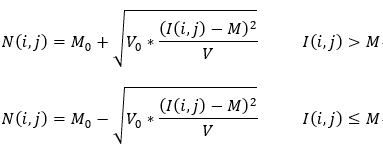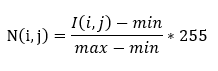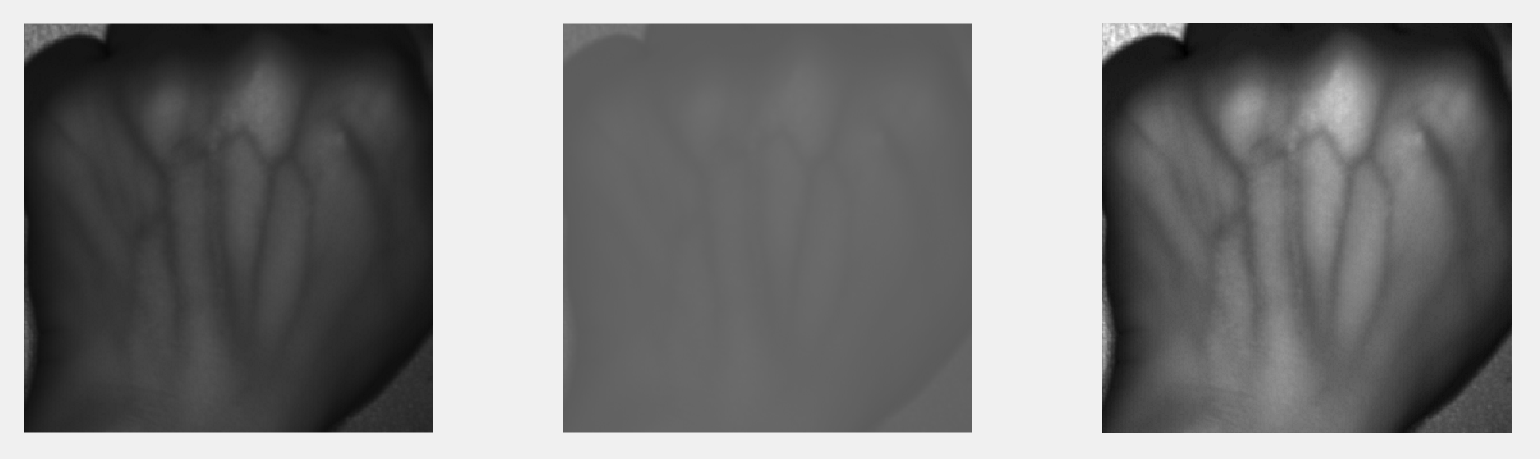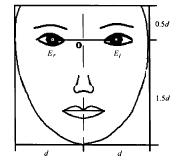图像处理中灰度归一化_灰度图像归一化处理 - CSDN
• 一般而言，对于灰度图像（或彩色通道的每个颜色分量）进行灰度归一化就是：使其像素的灰度值分布在0~255之间，避免图像对比度不足（图像像素亮度分布不平衡）从而对后续处理带来干扰。一种常见的图像归一化原理1是y=...
在许多图像处理系统中，对图像进行归一化都是必备的预处理过程。一般而言，对于灰度图像（或彩色通道的每个颜色分量）进行灰度归一化就是：使其像素的灰度值分布在0~255之间，避免图像对比度不足（图像像素亮度分布不平衡）从而对后续处理带来干扰。

一种常见的图像归一化原理1是y=(x-MinValue)/(MaxValue-MinValue)
其中x、y分别为归一化前、归一化后的值，MaxValue、MinValue分别为原始图像灰度的最大值和最小值。

源码示例（这里包含了三种方法：前两种是编程实现2，最后一种直接调用MATLAB函数来实现3，大家可以参考）：

oriImage = imread('XXXX.jpg');
grayImage = rgb2gray(oriImage);
figure;
imshow(grayImage);

originalMinValue = double(min(min(grayImage)));
originalMaxValue = double(max(max(grayImage)));
originalRange = originalMaxValue - originalMinValue;

% Get a double image in the range 0 to +255
desiredMin = 0;
desiredMax = 255;
desiredRange = desiredMax - desiredMin;
dblImageS255 = desiredRange * (double(grayImage) - originalMinValue) / originalRange + desiredMin;

figure;
imshow(uint8(dblImageS255));

% Get a double image in the range 0 to +1
desiredMin = 0;
desiredMax = 1;
desiredRange = desiredMax - desiredMin;
dblImageS1 = desiredRange * (double(grayImage) - originalMinValue) / originalRange + desiredMin;

figure;
imshow(dblImageS1);

% Another way to normalazation, which only calls MATLAB function
img3 = mat2gray(oriImage);
figure;
imshow(img3);

上述源码已在MATLAB 2014和MATLAB 2016上通过测试。

原始图片：运行后的效果：注意：如果在自己的图片上希望看到直观的效果，请模仿示例预先将测试图片的对比度调低一些，让图像中各个像素点的灰度分布范围不足 0-255。

参考资料：
http://blog.csdn.net/zengjiqin/article/details/50032893

http://blog.csdn.net/zengjiqin/article/details/50032893 ↩https://cn.mathworks.com/matlabcentral/newsreader/view_thread/297402 ↩http://blog.csdn.net/fx677588/article/details/53301740#comments ↩
展开全文matlab 数字图像处理
• 在许多图像处理系统灰度归一化是必备的预处理过程。以手背静脉图像为例。由于同一个手背在不同时间、不同光照下采集到的静脉图像在灰度分布上可能存在着很大的差异，图像的灰度分布很不集中，这些将直接影响到...
在许多图像处理系统中，灰度归一化是必备的预处理过程。以手背静脉图像为例。由于同一个手背在不同时间、不同光照下采集到的静脉图像在灰度分布上可能存在着很大的差异，图像的灰度分布很不集中，这些将直接影响到后续的特征提取和识别，因此要对手背静脉图像进行灰度归一化。

这里介绍两种灰度归一化的方法：均值方差归一化、灰度变换归一化。

1. 均值方差归一化

均值方差归一化是将不同时间、不同光照下采集到的图像转换到同一灰度均值和方差的标准图像。其公式为：式中，I(i,j)、 M、 V分别表示归一化前图像的灰度值、均值、方差，N(i,j)、M0、V0分别表示归一化后图像的灰度值、均值、方差。

2. 灰度变换归一化

灰度变换归一化是利用灰度拉伸的方法将原图像中的灰度分布扩展到具有整个灰度级的图像。例如，采集到的手背静脉图像是8位的灰度图像，应有256个灰度级，但往往由于采集时光照等因素的影响，图像的灰度级集中在一个或者几个灰度级区段，这时可以采用灰度拉伸的方法将图像扩展到256个灰度级。灰度变换归一化的公式为：式中，I(i,j)和N(i,j)分别表示原图像的灰度值、变换后图像的灰度值，min和max分别表示原图像的最小灰度值、最大灰度值。

参考文献：

 林喜荣，庄波，苏晓生等. 人体手背静脉血管图像的特征提取及匹配[J]. 清华大学学报：自然科学版. 2003,43(2). 164-167

 刘明奇，倪国强，陈小梅. 手背静脉图像预处理算法研究[J]. 光学技术，2007，33：255-256

以上述方法为依据，编写灰度归一化函数。

1. 均值方差归一化

function dstImage = grayNormalize1( srcImage, M0, V0 )
% 灰度归一化函数，将输入的图像转换为统一均值和方差的标准图像
% 输入参数：
%   srcImage -- 输入的图像
%   M0 -- 归一化后图像的均值
%   V0 -- 归一化后图像的方差
% 输出参数：
%   dstImage -- 输出的图像

% 编写时间：2018-12-6  编写人：gq

I = im2double(srcImage);
[m, n] = size(I);
J = zeros(m, n);
M = mean(mean(I)); % 输入图像的均值
V = std2(I); % 输入图像的方差

for i = 1 : m
for j = 1 : n
if I(i,j) > M
J(i,j) = M0 + sqrt( ( V0 * ( I(i,j)-M).^2 ) / V );
else
J(i,j) = M0 - sqrt( ( V0 * ( I(i,j)-M).^2 ) / V );
end
end
end

dstImage = uint8(J); 

2. 灰度变换归一化

function dstImage = grayNormalize2(srcImage)
% 灰度变换归一化，拉伸原图像的灰度级
% 输入参数：
%   srcImage -- 原灰度图像
% 输出参数：
%   dstImage -- 变换后的图像

% 编写时间：2018-12-17  编写人：gq

I = im2double(srcImage);  % 将原图像转换为浮点型数据

minValue = min(min(I));  % 获得原图像的最小灰度值
maxValue = max(max(I));  % 获得原图像的最大灰度值

[m, n] = size(I);
J = zeros(m, n);

for i = 1 : m
for j = 1 : n
J(i,j) = 255 * (I(i,j)-minValue) / (maxValue-minValue);
end
end

dstImage = uint8(J); 

调用上述两个灰度归一化函数。

close all;
clear;
clc;

% read an image into the workspace

% caculated by grayNormalize1
J1 = grayNormalize1(I, 100, 255);  % 设变换后图像的均值为100，方差为255

% caculated by grayNormalize2
J2 = grayNormalize2(I);

figure;
subplot(131), imshow(I);
subplot(132), imshow(J1);
subplot(133), imshow(J2); 

实验结果如下图所示。展开全文• 人脸的归一化包括几何归一化和灰度归一化，几何归一化分两步：人脸校正和人脸裁剪。而灰度归 一化主要是增加图像的对比度，进行光照补偿。 1.几何归一化 几何归一化的目的主要是将表情子图像变换为统一的尺寸，...
       在对人脸表情进行识别时，人脸的归一化处理是至关重要的一环，它涉及到下一步处理的好坏。
人脸的归一化包括几何归一化和灰度归一化，几何归一化分两步：人脸校正和人脸裁剪。而灰度归
一化主要是增加图像的对比度，进行光照补偿。
1.几何归一化
几何归一化的目的主要是将表情子图像变换为统一的尺寸，有利于表情特征的提取。具体步骤如下：
（1）标定特征点，这里用[x,y] = ginput(3)函数来标定两眼和鼻子三个特征点。主要是用鼠标动手标
定，获取三个特征点的坐标值。
（2）根据左右两眼的坐标值旋转图像，以保证人脸方向的一致性。设两眼之间的距离为d，其中点为O。
（3）根据面部特征点和几何模型确定矩形特征区域，以O为基准，左右各剪切d，垂直方向各取0.5d和
1.5d的矩形区域进行裁剪。
（4）对表情子区域图像进行尺度变换为统一的尺寸，更有利于表情特征的提取。把截取的图像统一规格
为90*100的图像，实现图像的几何归一化。
面部几何模型如下图：2.灰度归一化
灰度归一化 主要是增加图像的亮度，使图像的细节更加清楚，以减弱光线和光照强度的影响。
具体代码如下：
figure(1),imshow(C);
C=double(C);
figure(2),imshow(image/255);
title('Lighting compensation');%光照补偿
[x,y] = ginput(3);    %%1 left eye, 2 right eye, 3 top of nose
cos = (x(2)-x(1))/sqrt((x(2)-x(1))^2+(y(2)-y(1))^2);
sin = (y(2)-y(1))/sqrt((x(2)-x(1))^2+(y(2)-y(1))^2);
mid_x = round((x(1)+x(2))/2);
mid_y = round((y(2)+y(1))/2);
d = round(sqrt((x(2)-x(1))^2+(y(2)-y(1))^2));
rotation = atan(sin./cos)*180/pi;
img = imrotate(image,rotation,'bilinear','crop');
figure(3), imshow(img);%人脸校正
[h,w] = size(img);
end
end
end
end
I1 =[];
I2 =[];
I2(:,:) = imresize(I1,[90 100]);
figure(4),imshow(I2,[]);%人脸裁剪


展开全文• 在统计学归一化的具体作用是归纳统一样本的统计分布性。归一化在0-1之间是统计的概率分布，归一化在-1--+1之间是统计的坐标分布。 即该函数在(-∞,+∞)的积分为1 例如概率的密度函数就满足归一化条件 ...

在统计学中，归一化的具体作用是归纳统一样本的统计分布性。归一化在0-1之间是统计的概率分布，归一化在-1--+1之间是统计的坐标分布。

即该函数在(-∞,+∞)的积分为1

例如概率中的密度函数就满足归一化条件

归一化函数举例：

线性函数转换如下

y=(x-MinValue)/(MaxValue-MinValue)

说明：x、y分别为转换前、后的值，MaxValue、MinValue分别为样本的最大值和最小值。

数函数转换如下

y=log10(x)

说明：以10为底的对数函数转换。

反正切函数转换如下

y=atan(x)*2/PI

opencv实现归一化：

cvMinMaxLoc(mat_low_double,&minvalue,&maxvalue);cvConvertScale(mat_low_double,mat_low_double,1,-minvalue);cvConvertScale(mat_low_double,mat_low_double,255/(maxvalue-minvalue),0);//归一化


展开全文• cv::normalize(src, dst, 0, 255, NORMAL_MINMAX) 将灰度拉伸到0~255之间 cv::normalize(src, dst, 0, 1, NORMAL_MINMAX) 将灰度归一化到0~1之间
• 一共有两种归一化操作 ** 把任意数值归一化0-255之间： * oriImage = imread(‘XXXX.jpg’); grayImage = rgb2gray(oriImage); figure; imshow(grayImage); originalMinValue = double(min(min(grayImage))...
• 归一化： 就是要把需要处理的数据经过处理后（通过某种算法）限制在你需要的一定范围内。...在许多图像处理系统，对图像进行归一化都是必备的预处理过程。一般而言，对于灰度图像（或彩色通道的每个颜...
• I=imread('D:\project1.jpg'); I=im2double(I); [M,N]=size(I); figure(1); imshow(I); title('source'); figure(2); [H,x]=imhist(I,32); stem(x,(H/M/N),'.'); title('source1'); %增加对比度 ......matlab 图像识别
• 1.傅里叶反变换后得到图像类型是“real”，但是处理图像是“byte”类型。 于是再网上白嫖了： convert_image_type( Image : ImageConverted : NewType : ) 函数作用： 转换图像类型 如果将大的灰度范围类型转换为小...计算机视觉 opencv
•图像处理
• 二：图像归一化处理 图像归一化最常见的就是最大最小值归一化方法，公式如下： OpenCV中实现图像最大与最小值归一化的函数... alpha=None, // 归一化中低值 min beta=None, // 归一化中的高值max norm_type=No...
• 图像直方图归一化 图像直方图概念： 图像直方图是反映一个图像像素分布的统计表，其实横坐标代表了图像像素的种类，可以是灰度的，也可以是彩色的。纵坐标代表了每一种颜色值在图像中的像素总数或者占所有像素个数的...MATLAB 数字图像处理 图像直方图
• 图像归一化是一个像素级操作，其作用是在不改变脊线和谷线结构的清晰度的情况下减少脊谷灰度值的变化，方便后续的处理步骤。对于指纹数据库来说，在进行完图像归一化操作后，图像具有相同的灰度均值和灰度方差，减小...算法 计算机视觉
• 一、图像归一化的好处：1、转换成标准模式，防止仿射变换的影响。2、减小几何变换的影响。3、加快梯度下降求最优解的速度。二、图像归一化的方法1、线性函数转换，表达式如下：y=(x-MinValue)/(MaxValue-MinValue)...图像归一化
• 通过对图像的RGB色彩空间进行归一化处理，在某些情况下是去除光照和阴影影响的一种简单和有效的方法。 假设RGB代表原图像某点的像素值，rgb表示归一化之后的值，则 r = R / (R+G+B)；g = G / (R+G+B)；b = B /...
• matlab图像处理为什么要归一化和如何归一化， 一、为什么归一化 1.  基本上归一化思想是利用图像的不变矩寻找一组参数使其能够消除其他变换函数对图像变换的影响。也就是转换成唯一的标准形式以抵抗仿射变换 　...
• 利用OpenCV 对图像像素进行操作，计算归一化直方图，并在窗口以图形的方式显示出来。归一化直方图 opencv
• 在输入路径下输入待处理图片的所在文件夹路径，输出路径下输入保存图片的文件夹路径，选择灰度化或归一化，也可同时选择，输入归一化的宽和高的大小，点击开始处理处理图片如下： 处理完毕界面截图，...opencv
...